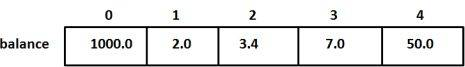# 数组

## 数组

C++ 提供了一种数据结构，数组，它存储了一个固定大小的相同类型的有序集合的元素。一个数组通常被用来存储大量的数据，但往往将数组看作是一个相同类型的变量的集合。

## 声明数组

``    type arrayName [ arraySize ];``

``    double balance;``

## 初始化数组

``    double balance = {1000.0, 2.0, 3.4, 17.0, 50.0};``

``    double balance[] = {1000.0, 2.0, 3.4, 17.0, 50.0};``

``    balance = 50.0;``## 访问数组元素

``    double salary = balance;``

``````    #include <iostream>
using namespace std;

#include <iomanip>
using std::setw;

int main ()
{
int n[ 10 ]; // n is an array of 10 integers

// initialize elements of array n to 0
for ( int i = 0; i < 10; i++ )
{
n[ i ] = i + 100; // set element at location i to i + 100
}
cout << "Element" << setw( 13 ) << "Value" << endl;

// output each array element's value
for ( int j = 0; j < 10; j++ )
{
cout << setw( 7 )<< j << setw( 13 ) << n[ j ] << endl;
}

return 0;
}``````

``````    ElementValue
0  100
1  101
2  102
3  103
4  104
5  105
6  106
7  107
8  108
9  109``````

## 详细的 C++ 数组

App下载